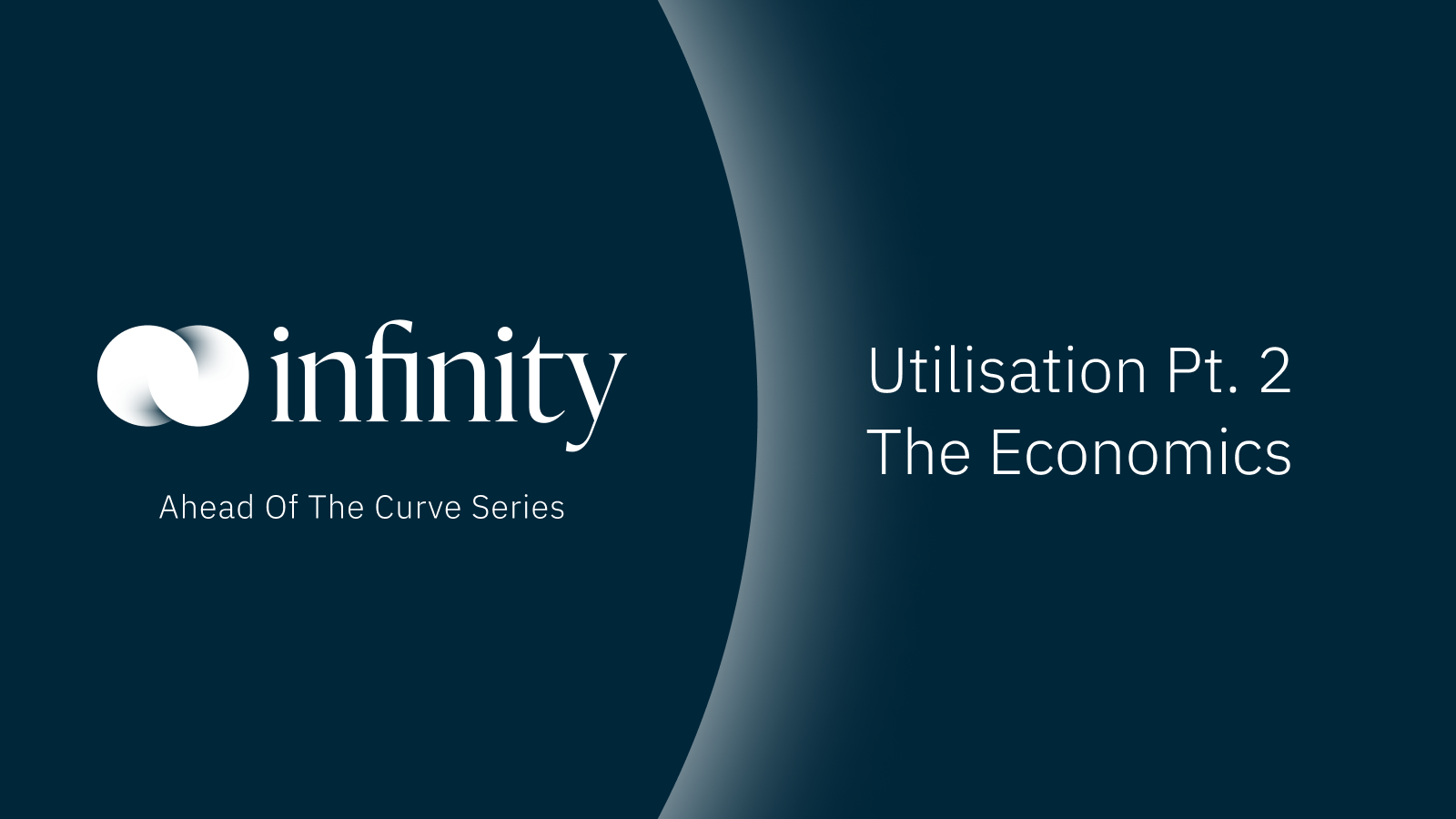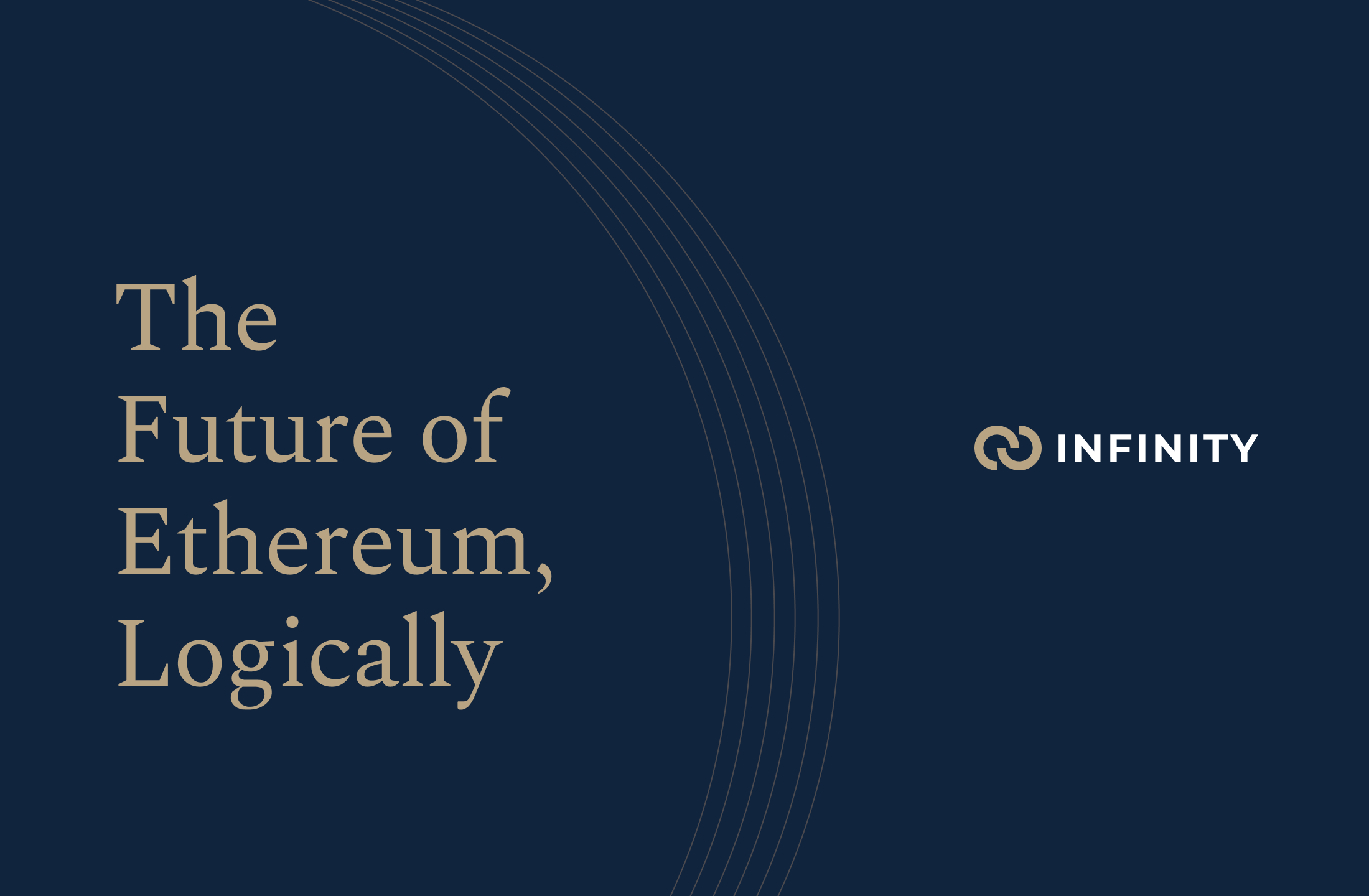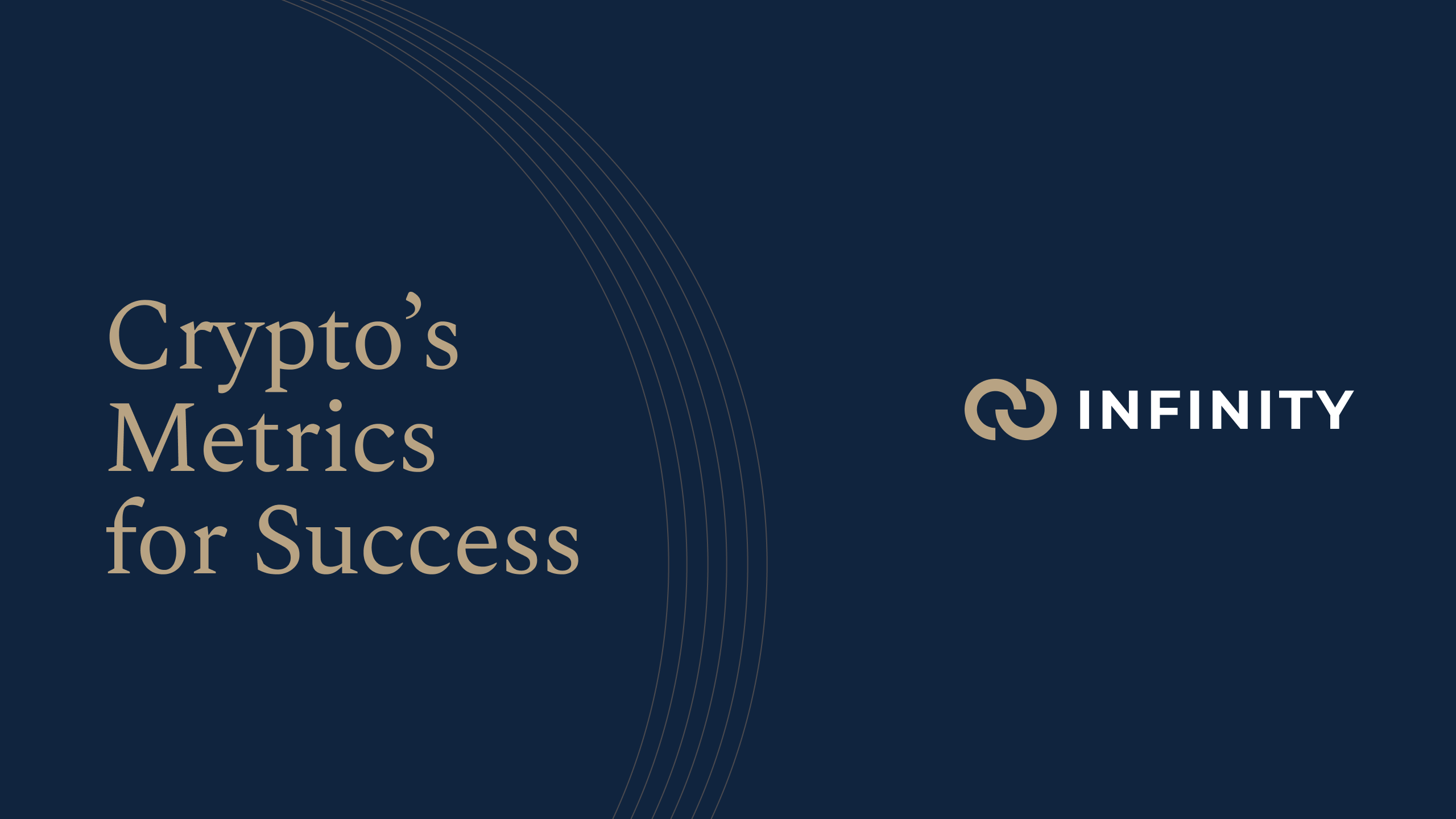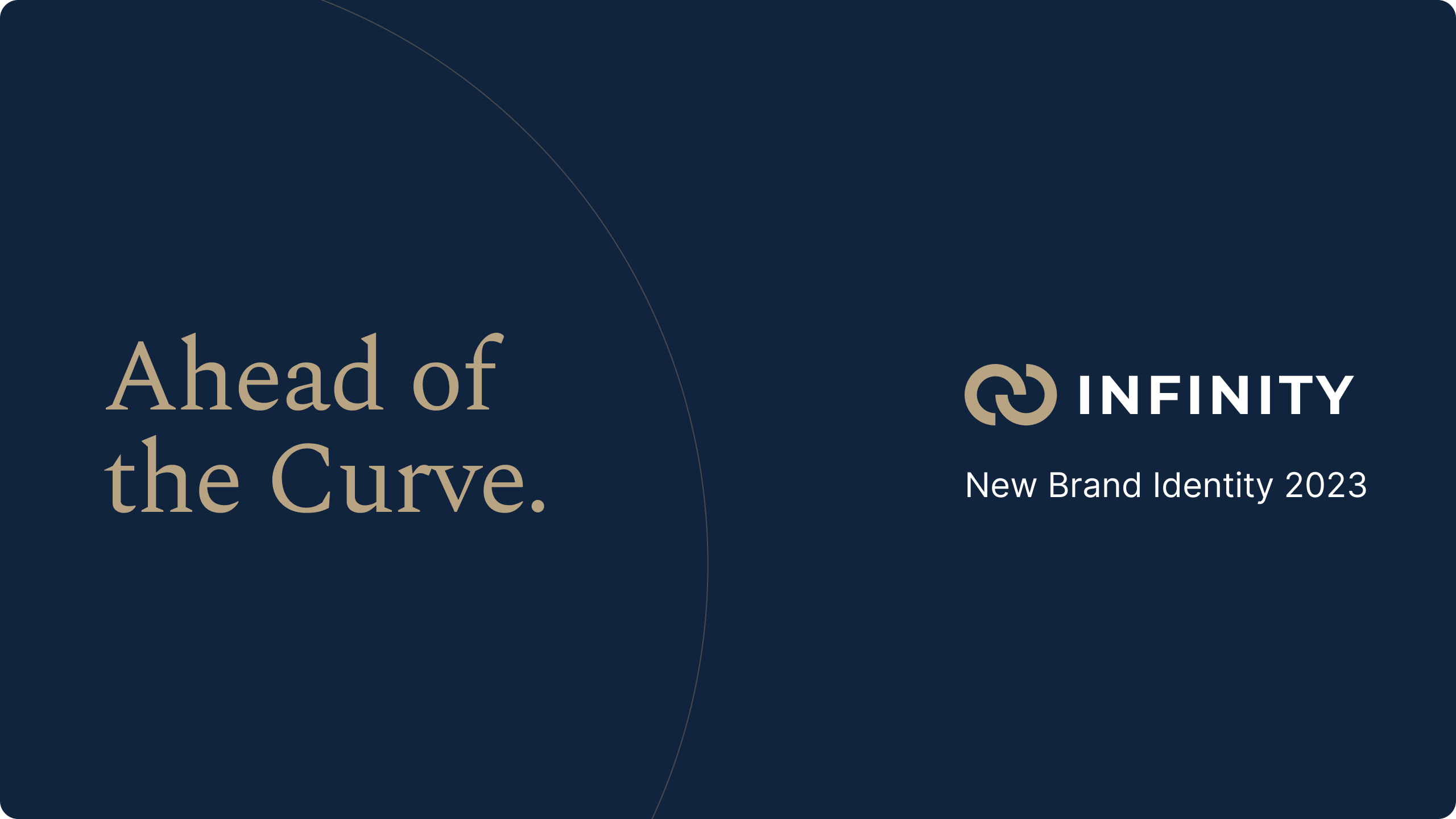# Utilisation — How do we electrify DeFi? (pt. 2)

Jun 9, 2023TLDR;

• Infinity Exchange is setting out to create a borrowing and lending protocol that always operates at 100% Utilisation.
• With this new mechanic, Infinity matches a quantity of lenders with the same quantity of borrowers.

In Part 1, we introduced the concept of Utilisation, its relationship with Interest rates, and the Goldilocks dilemma resulting in wide spreads that plague the current borrowing and lending primitives (DeFi 1.0). As a follow up, we discuss the inefficiencies (deadweight loss) resulting from uneven notional / principal matching protocols and explain how Infinity Exchange is going to revolutionize DeFi. This article accompanies (What is Utilisation — pt. 2).

Let’s start off by affirming the fundamental concept of Supply and Demand.

If we plot a graph (screenshot from the video above), with

• ‘Y’ or vertical axis as the Price (i.e. Interest rate);
• ‘X’ or horizontal axis as the Quantity (i.e. Borrowers and Lenders);
• Upward sloping Supply (i.e. Lending) curve; and
• Downward sloping Demand (i.e. Borrowing) curve.

The point where the Supply and Demand curve ‘intersects’ (i.e. the circle in the middle of the graph) represents an equilibrium of Lending and Borrowing, where the notional and principal of lenders and borrowers would be matched / identical. From the above example, that would be a quantity of 100 at an Interest rate of 5%.

##### Recall from Part 1
• As Utilisation increases, Interest rate increases; and
• As Interest rate increases, Lenders are incentivised to lend more.

From the above graph, if we increased the Interest rate to 6% (i.e. moving ‘up’ the Y or vertical axis), what would result?

1. We move ‘up’ (i.e. to the right) on the upward sloping Supply curve. The quantity of lenders increases to (say) 130 (or 30 more than equilibrium of 100).
2. We move ‘up’ (i.e. to the left) on the downward sloping Demand curve. The quantity of borrowers decreases to (say) 60 (or 40 less than equilibrium of 100).
3. This results in an increase in the Interest rate with an (i) an increase in Supply (of lenders) and (ii) a decrease in Demand (from borrowers).

In our previous article [Utilisation (pt. 1)], we demonstrated that lending and borrowing protocols such as Aave and Compound operated with an uneven notional / principal matching of lenders and borrowers, leading to a deadweight loss or wide bid offer spreads, which made them untenable for usage by institutional ‘traditional’ finance.

We set out to create a borrowing and lending protocol that always operated at 100% Utilisation.

Based on the above example, Infinity Exchange would operate at the Quantity of 100 with an Interest rate of 5%. That is, we would operate with notional / principal matching. In contrast, the current ecosystem sets Interest rates in a deterministic and inefficient manner. We are here to set the record straight.

## The evolution from DeFi 1.0 to 2.0

The ideal point we want to achieve is a perfect state of matching lenders and borrowers.

However, the current ecosystem allows an uneven amount of lenders and borrowers. Based on the graph above (not to scale), where the Interest rate is 6%, the quantity of borrowers at 60 does not equal the quantity of lenders at 80.

While borrowers pay a 6% Interest rate, lenders would only receive 4.5%, given the Utilisation rate of 75% (calculated as 60 borrowers divided by 80 lenders; or simply 6% multiplied by 75%). As such, the difference between what borrowers pay and lenders receive is a 1.5% deadweight loss.

Recall, deadweight loss is baked into how DeFi 1.0 is built.

Let’s apply some ‘microeconomics’ and illustrate this with some colours in the above graph.

1. Borrower surplus highlighted in the yellow area.
2. Lender surplus highlighted in the red area.
3. The large (1.5% bid offer spread) deadweight loss highlighted as the blue area.
4. In all three cases, there is a large amount of market trading turnover or volume that is simply ‘lost’ as a result of how their mechanic is built.

In a proper and functioning market, we would have 100 lenders and 100 borrowers, such that the deadweight loss would not occur and, as a result, lenders and borrowers would be much happier with the Interest rate, as they each pay and receive the same 5%.

## One small tweak from DeFi 1.0, one giant leap to DeFi 2.0

We have illustrated the fundamental difference of DeFi 1.0 vs. DeFi 2.0.

Don’t get us wrong: DeFi 1.0 works, but it is simply inefficient.

With DeFi 2.0, we match the quantity of lenders with the same quantity of borrowers. This ensures both lenders and borrowers get the best Interest rate possible. With that, we asked ourselves,

What could happen to the overall size of the DeFi market with more efficient Interest rates?

We pulled up DeFi Llama and looked up all the main DeFi protocols in the current ecosystem.

Specifically, the two biggest incumbent lending protocols (Aug. 2022) are:

1. Aave with \$6.8B in total value locked (TVL); and
2. Compound with \$2.9B in TVL.
3. Now, if we combined their TVLs, this would be a (rounded up) total of \$10B.

As illustration, if the current size of TVL is \$10B and our previous graph shows how their mechanic includes a deadweight loss baked in, then we can imply that if Infinity were to bring the current market to an equilibrium state, the market would be bigger, much bigger.

## Infinity Exchange. DeFi 2.0

We have illustrated why the current lending and borrowing protocols are great as a proof of concept 1.0, whereby they drew a couple of lines and applied a relationship between Utilisation and Interest rates, but at the end of the day, we similarly illustrate why their mechanic is inefficient.

We are building towards a state of DeFi 2.0 where all borrowers and lenders can magnanimously access the same type of traditional ‘institutional’ finance experience. If we can do this properly, we have no doubt that Infinity will deliver an eye-wateringly increase in the size of the current market.

DeFi 1.0. Spilt milk under the bridge.

Now, onwards and upwards. How will we get to DeFi 2.0?

Our next article introduces our notional / principal matched Two Market Model (TMM).

Stay tuned.

### Our Social Media

Website: www.infinity.exchange
Discord: https://discord.com/invite/cb3DW9zMtB
Telegram: https://t.me/infinity_exchange
Medium: https://medium.com/infinityexchange

Get in touch: community@infinity.exchange or investors@infinity.exchange

### Related ArticlesIn this article, we explore the relationships between ETH Staking, and ETH Floating/Fixed Interest rates. How are they related? How are they different? Let's find out...

June 9, 2023We went in search of the metrics that Crypto holds itself accountable to, and voila, they didn't exist. We then set out to map out what would be a good starting point.

June 9, 2023Ushering in a new era of transparency and stability for the future of Crypto

June 9, 2023